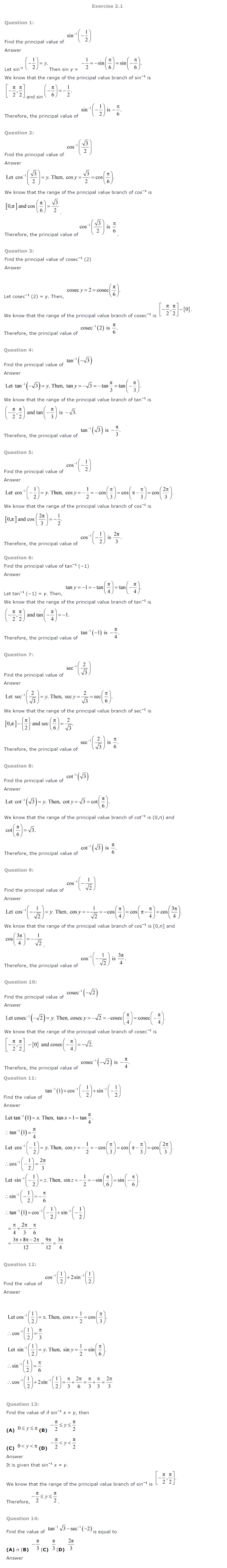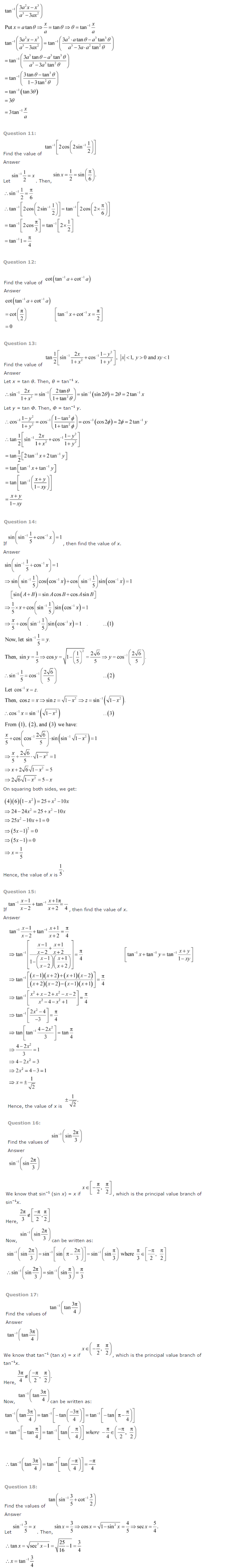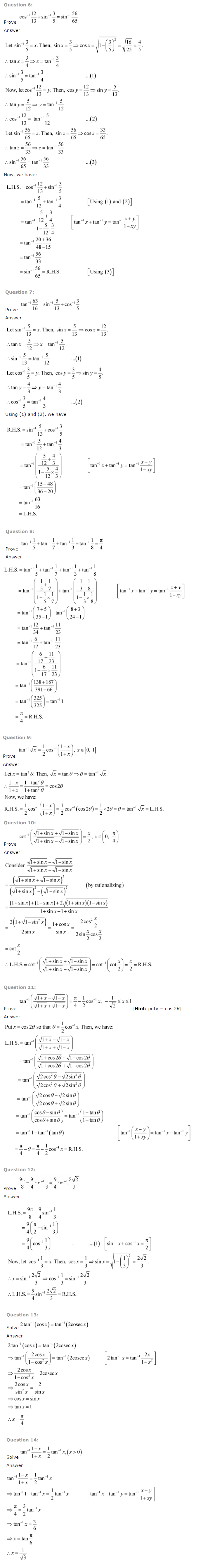NCERT Books » NCERT Solutions PDF Download » NCERT Solutions For Class 12 PDF Download » NCERT Solutions For Class 12 Maths » NCERT Solutions Class 12 Maths Ch 2 Inverse Trigonometric Functions

# NCERT Solutions Class 12 Maths Ch 2 Inverse Trigonometric Functions

## NCERT Solutions Class 12 Maths Ch 2 Inverse Trigonometric Functions PDF Download

The NCERT Solutions Class 12 Maths Ch 2 Inverse Trigonometric Functions will help in preparation of Class 12 board exams, achieving rank in JEE (Main and Advanced) and scoring marks in other exams. The NCERT Solutions Class 12 Maths Ch 2 Inverse Trigonometric Functions has Ex 2.1 Introduction, Ex 2.2 Basic Concepts, Ex 2.3 Properties of Inverse Trigonometric Functions.

#### Topics and Exercises in NCERT Solution Class 12 Maths Chapter 2 Inverse Trigonometric Functions:

• Ch 2 Inverse Trigonometric Functions
• Ex 2.1 Introduction
• Ex 2.2 Basic Concepts
• Ex 2.3 Properties of Inverse Trigonometric Functions

### Download NCERT Solutions Class 12 Maths Ch 2 Inverse Trigonometric Functions